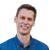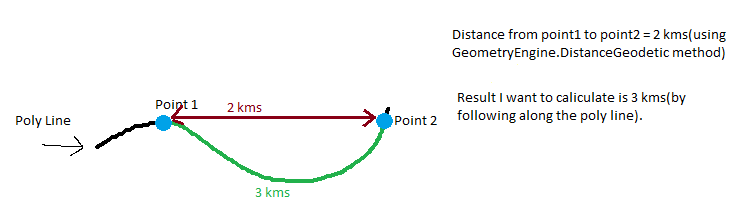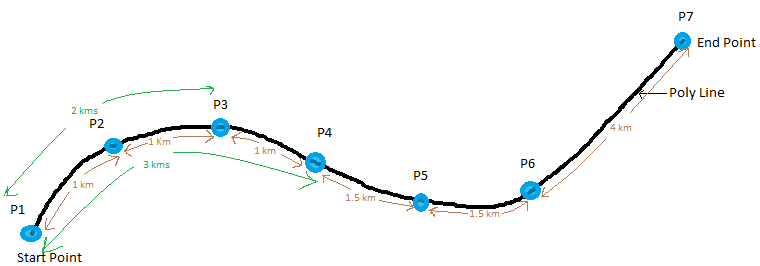# Identify the distance between two co-ordinates (Lat and Long) on a polyline

1009
4
02-24-2019 11:16 PMNew Contributor II

Good day team

We are trying to calculate the distance between two co-ordinates on a polyline by passing point 1 and point 2 as a parameter by using the following code:

``GeometryEngine.DistanceGeodetic(startPoint, endPoint, LinearUnits.Meters, AngularUnits.Degrees, GeodeticCurveType.Geodesic).Distance‍‍‍‍``

The result of the above method is only returning the straight line distance instead of considering curves etc. This really pushing our distance calculation off. Could you please advise the best suitable method for the above purpose.

4 RepliesbyEsri Frequent Contributor

The result of the above method is only returning the straight line distance instead of considering curves etc

I'm not sure what you mean by this? The above code would return the shortest distance between the two points, which will be a straight line (but following the curvature of the earth, rather than going through the earth's crust). However that straight line might not look straight on a projected map and appear curved due to the distortion caused by the projection.

Could you give an example of two coordinates, and the distance you're getting vs. what you'd expect?New Contributor II

Thank you for the response

Following are my requirements, I'm developing a WPF application using ARCGis SDK for .Net and c# programming.

Requirement 1need to find the distance between two points by following a poly line.

Here i'm having a Poly line p and point1 and point2 are the points on the polyline p, now want to calculate the distance between Point1 and Point2 by following the polyline which is 3 kms, but GeometryEngine.DistanceGeodetic methods returns the shortest and linear distance between the points which is 2 kms. So please suggest a way to achieve this.Requirement 2 : need to sort all the points on a polyline by distance(from starting point) in ascending.

Here i'm having a poly line with 7 points as p1,p2,p3,p4,p5,p6,p7. Distance between the points on the polyline as follows

P1 to P2 = 1 kms

P2 to P3 = 1 kms

P3 to P4 = 1 kms

P4 to P5 = 1.5 kms

P5 to P6 = 1.5 kms

P6 to P7 = 4 kms

----------------------------------

Total polyline Distance = 10 kms

So, i want to arrange these points in ascending order based on their distance from start point of the poly line,i.e,.

Point    Distance(from start point)

P1         0 kms

P2         1 kms

P3         2 kms

P4         3 kms

P5         4.5 kms

P6         6 kms

P7         10 kmsbyEsri Frequent Contributor

If you want the length of a line, and not the distance between two points, use the GeometryEngine.LengthGeodetic method instead.

Or you can sum up the distances between P1->P2, P2->P3, P3-P4 etc.New Contributor II

Thank you for the response Morten Nielsen,

As per your suggestion we would like to use the GeometryEngine.LengthGeodetic method to find the length of a polyline between two points. Please note that GeometryEngine.LengthGeodetic expects poly line as a parameter. Could you please explain how do we split the above long poly line into parts at each points as small poly lines or links so that we can calculate the distance for each small poly line or link.